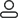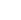LanguageLogin|Sign up
Blog >> Blog Details Page
Characteristic impedance of printed circuit boards
Posted:10:50 AM January 08, 2019 writer: G

1. Characteristic impedance

1.1. Resistance

When an alternating current flows through a conductor, the resistance it receives is called Impedance, which is Z, and the unit is Ω. The resistance at this time is different from the resistance encountered by the direct current. In addition to the resistance of the resistor, there is also a problem of resistance between the inductive reactance (XL) and the capacitive reactance (XC). To distinguish the resistance of the DC, the resistance encountered by the AC is called the impedance (Z). Z=√ R2 +(XL -XC)2

1.2. Impedance (Z)

In recent years, the improvement and application of IC integration has become more and more high in signal transmission frequency and speed. Therefore, in the printed circuit board wire, after the signal transmission (emission) is high to a certain value, the printed circuit board wire itself is received. The effect of this results in severe distortion or complete loss of the transmitted signal. This shows that the "thing" that the PCB wire "circulates" is not the current, but the energy transfer of the square wave signal or pulse.

1.3. Characteristic impedance control (Z0)

The resistance to the above-mentioned "signal" transmission is also referred to as "characteristic impedance", and the representative symbol is Z0.

Therefore, the problem of "on", "off" and "short circuit" on the PCB wire is not enough, and the characteristic impedance problem of the wire is also controlled. That is to say, the transmission line for high-speed transmission and high-frequency signal transmission is much stricter in quality than the transmission line. It is no longer an “open/short” test pass, or the notch or burr does not exceed 20% of the line width and can be received. The characteristic impedance value must be determined. This impedance should also be controlled within the tolerance. Otherwise, it must be scrapped and must not be reworked.

2. Signal transmission and transmission line

2.1. Signal transmission line definition

(1) According to the principle of electromagnetic waves, the shorter the wavelength (λ), the higher the frequency (f). The product of the two is the speed of light. That is, C = λ.f = 3 × 1010 cm / s

(2) Any component, despite its high signal transmission frequency, will have a very high transmission frequency or time delay after transmission through the PCB conductor. Therefore, the shorter the wire length, the better.

(3) It is advantageous to increase the PCB wiring density or shorten the wire size. However, as the component frequency increases, or the pulse period shortens, the wire length approaches a certain range of signal wavelength (speed), and when the component is transmitted on the PCB wire, significant "distortion" occurs.

(4) IPC-2141 3.4.4 proposes that when the signal is transmitted in the wire, if the wire length is close to 1/7 of the signal wavelength, the wire at this time is regarded as the signal transmission line.

(5) Example: The signal transmission frequency (f) of a component is 10 MHz, and the length of the wire on the PCB is 50 cm. Should special impedance control be considered?

Solution: C = λ.f = 3 × 1010 cm / s

λ=C/f=(3 × 1010 cm/s)/(1 ×107 /s )=3000cm

Wire length / signal wavelength = 50 / 3000 = 1 / 60

Because: 1/60<1/7, this wire is a normal wire, and it is not necessary to consider the characteristic impedance problem.

In the theory of electromagnetic waves, the Maxwell formula tells us that the propagation velocity VS of a sinusoidal signal in a medium is proportional to the speed of light C and inversely proportional to the dielectric constant of the transmission medium.

VS = C / √ εr

When εr =1, the signal transmission reaches the propagation speed of light, ie 3 × 1010 cm/s.

2.2. Transmission rate and dielectric constant

Signal transmission speed of different plates at 30MHZ

Dielectric material Tg( °C ) Dielectric constant Signal transmission speed (m/μs)

Vacuum / 1.0 300.00

PTFE / 2.2 202.26

Thermoset poly (diethyl ether) 210 2.5 189.74

Cyanate resin 225 3.0 173.21

PTFE resin + E glass cloth / 2.6 186.25

Cyanate resin + glass cloth 225 3.7 155.96

Polyimide + glass cloth 230 4.5 141.42

Quartz / 3.9 151.98

Epoxy glass cloth 130±5 4.7 138.38

Aluminum / 9.0 100.00

As can be seen from the above table, as the dielectric constant (εr) increases, the transmission speed of the signal in the dielectric material decreases. To obtain a high signal transmission speed, a high characteristic impedance value is required; a high characteristic impedance must be selected from a low dielectric constant (εr) material; a dielectric constant (εr) of Teflon is minimized, and transmission is required. The fastest.

The FR-4 sheet is composed of an epoxy resin and an E-grade glass cloth, and has a dielectric constant (εr) of 4.7. The signal transmission speed is 138 m/μs. Changing the resin system makes it easier to change the dielectric constant (εr).

773 2 0 1
• PCB
Prototype
• PCB
Assembly
• SMD
Stencil
 Dimensions: (mm) × Quantity: (pcs) 5 5 10 15 20 25 30 40 50 75 100 120 150 200 250 300 350 400 450 500 600 700 800 900 1000 1500 2000 2500 3000 3500 4000 4500 5000 5500 6000 6500 7000 7500 8000 9000 10000 Other Quantities:(quantity*length*width is greater than 10㎡) OK Layers: 1 2 4 6 8 10 12 Thickness: 0.6 mm 0.8 mm 1.0 mm 1.2 mm 1.6 mm 2.0 mm 2.5 mmQuote now